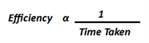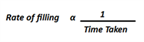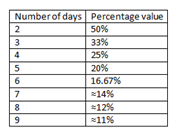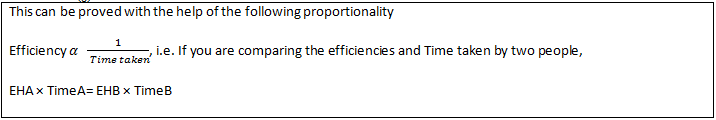# Time And Work Introduction

Time and Work is important topic in the Quantitative Aptitude section of the CAT exam. The questions related to time and work are asked every year and hold a good weightage as the various applications of time and work (like pipes and cisterns) are also included. So, to help the candidates prepare the topic well, here is a detailed introduction of Time and Work along with easy illustrations.

## Introduction: Time And Work

Work is defined as something which has an effect or outcome; often the one desired or expected. The basic concept of Time and Work is similar to that across all Arithmetic topics, i.e. the concept of Proportionality.

Efficiency is inversely proportional to the Time taken when the amount of work done is constant.This can be used to compare efficiencies and Time taken across different groups In Time-Speed-Distance, efficiency is replaced by Speed; i.e. Speed is inversely proportional to Time when the Distance is constant.

Pipes and Cisterns are just an application of Time and Work. Concept wise, it is one and the same. In the above proportionality, Efficiency is replaced by Rate of filling. The equation in this case becomesThe concept of the percentage of work done can be used to solve most of the related questions.

A few basic points one needs to know to use the percentage concept are: When it is said that someone has done a work, it means he has done 100 % of the work. Hence, if A finishes work in 4 days, it means- in 4 days he will do 100% of the work. Hence, in one day he finishes 25% (100/4) of the work. Similarly, in 3 days he finishes 75 % of the work.

## Time and Work Shortcut Tricks:

### Table of commonly used numbers:The complete work can also be considered as 1 unit. Then if A takes 4 days to finish a work, it means he can finish 1/4th of the work in 1 day.

## Illustrations:

Here are some basic questions to illustrate time and work shortcut tricks.

Example 1)

Rahim can finish the work in 10 days and Ram can finish the same work in 40 days. If Ram and Rahim both work together then what is the total number of days taken?

Solution:

The problem can be solved in two different approaches.

### Approach 1: Using Fractions

Ram can finish the work in 10 days i.e. in one day he will do 1/10th of the work.

Rahim can finish the work in 40 days i.e. in one day he will do 1/40th of the work.

So, in one day, both working together can finish= (1/10) + (1/40) = 5/40 = 1/8th of the work. So, to complete the work they will take 8 days.

### Approach 2: Using Percentage (Shortcut- Recommended)

Rahim can finish 100 % of work in 10 days i.e. in one day he finishes 10% of the work.

Ram can finish 100% of the work in 40 days i.e. in one day he finishes 2.5 % of the work.

So, working together, in a single day they can finish 12.5% of the work. So, to complete 100% of the work, it will take 100/12.5 = 8 days.

Example 2)

Ravi can do a job in 10 days. Raman can do the same job in 20 days. They together start doing the job but after 4 days Raman leaves. How many more days will be required by Ravi to complete this job alone?

Solution:

Ravi can finish a job in 10 days i.e in one day he can finish 10 % of the job.

Raman can finish the same job in 20 days i.e. in one day he can finish 5 % of the job.

So, working together, in a day they can do 10 + 5 = 15 % of the job.

In the 4 days, if they worked together, they would have finished 4 × 15% = 60% of the job.

So, job left = 100 – 60 = 40%. This work has to be done by Ravi who does 10 % of the job in a day. So, to finish the remaining 40%, he will take 40/ 10 = 4 more days.

## Introduction: Negative Work

Negative work increases the Time in which a work is to be completed. This application can be extended to cases involving Pipes and cisterns. Suppose there are two pipes in a Cistern. Pipe A is used to fill the Cistern and Pipe B is used to empty the Cistern. Here we say that Pipe B and Pipe A are working against each other. When a leak is developed in the Cistern, the leak forms the component of negative work, which slows down the completion of the task (in this case, the filling of the Cistern)

The following questions will explain the concept better.

## Illustrations:

Example: 1)

Pipe A can fill a tank in ‘an’ hours. On account of a leak at the bottom of the tank, it takes thrice as long to fill the tank. How long will the leak at the bottom of the tank take to empty a full tank, when pipe A is kept closed?

a) (3/2)hours

b) (2/3)hours

c) (4/3)hours

d) (3/4)hours

Solution:

Method 1: Using variables

The pipe can fill (1/a)^th of the tank in an hour. Because of the leak, it can only fill 1/3a of the tank per hour. Let X be the Time in which the leak can completely empty the tank, hence 1/x=1/a-1/3a

= x=3a/2hrs. Option (a)

Method 2: Using Numbers (Shortcut)

Assume a value for “a”- say 10 hours. Because of the leak, it will take 30 hours. Now, this means that in these 30 hours, the filling will occur at a rate of 3.33% and the leaking will slow down the process by 6.66% every hour Thus, the Time taken to empty a full tank= 100/6.66= 15 hours.

Answer: Only option (a) satisfies this value.

Example:2)

A Cistern has three pipes A, B, and C. Pipe A can fill a Cistern in 10 hrs, Pipe B can fill a Cistern in 5 hrs while Pipe C can empty the Cistern in 20 hrs. If they are switched on at the same Time; in how many hours will the Cistern be filled?

Solution:

In one hour Pipe A can fill 100/10= 10% of the Cistern.

In one hour Pipe B can fill 100/5= 20 % of the Cistern.

In one hour Pipe C can empty 100/20= 5 % of the Cistern.

If all three are working together, (10 + 20 – 5) =25% of the Cistern will get filled in one hour, so it will take 4 hrs for the Cistern to fill.

Example:3)

A tank can be filled by tap A in 6 hrs and by tap B in 3 hrs. But when they are open simultaneously to fill an empty tank they take 3 hrs more than their normal Time. A hole is later discovered as the reason for the delay. Find the Time taken by the hole to empty the tank if it is completely filled.

Solutions:

Tap A takes 6 hrs to fill, so in one hour it will fill 16.67 % of the tank. Tap B takes 3 hrs to fill, so in one hour it will fill 33.33% of the tank. Together they will fill 16.67% + 33.33% = 50 % of the tank. They should take 100/50= 2 hrs to fill the tank. But they take 3 hrs more, because of the hole; they totally take 5 hrs to fill i.e. they fill 20% in an hour.

This is possible if the hole empties 50-20 = 30 % of the tank in an hour. So to completely empty the full tank; the hole will take 100/30 hrs = 3.33 hrs = 3 hrs and 20 mins.

## Inverse Proportionality of Efficiency and Time Taken

If the product of the two variables is always constant, the two are said to be inversely proportional.

Efficiency and Time taken are inversely proportional implies that If A is twice as good as B then A will take half the Time that B will take.

If the efficiencies are in the ratio m: n then Time taken will be in the ratio, n: m. i.e. If A is thrice is as good as B then A will take (1/3)^rdof the Time.## Illustrations:

Example 1:

C takes 30 days to finish work and A is thrice as good as C and twice as good as B. If A, B, and C work together, then how many days they will take to finish the work?

Solutions:

Approach 1:

C takes 30 days to finish the work. Since A is thrice as good as C, A will take 10 days to finish the work Since A is twice as good as B, B will take 20 days to finish the work. Together A, B, and C will take 1/(1/10+1/20+1/30) = 5.4545 days.

Approach 2: Using Percentages (Shortcut)

1 day = 3.33% work by C

1 day= 10% work by A

1 day= 5% work by B

Together, in one day = 18.33% of work. Thus they will take 100/18.33 days= 5.4545

Example 2:

A is twice as efficient as B. if they take 6 days to finish a certain job together, how much Time will they individually take to complete the work?

Solution:

Using the percentage approach It is given that A is twice as efficient as B. If they are finishing the work in 6 days, then in one day they will do 100/6 % of work= 16.66% as A is twice as efficient, work done by A in one day will be twice the work done by B. So work done by A in one day = 2/3 * 100/6 % = 100/9 %= 11.11% Work done by B in one day = 100/18 %= 5.55%. So, A will take 9 days to finish the job individually while B will take 18 days.

For more questions on this topic, visit time and work questions.

Stay tuned with BYJU’S to know the latest CAT notifications along with the pattern and various CAT sample papers to strategize a proper preparation plan and learn effectively.

With this Time and Work introduction and shortcuts, the CAT candidates can easily learn the concept and solve the questions accurately. It is suggested to take numerous CAT mock tests to be able to analyze the preparation level and strategies accordingly.

To learn more important CAT exam topics, stay tuned with BYJU’S. Also, learn more effectively with visualization and know various shortcut techniques from different engaging video lessons.